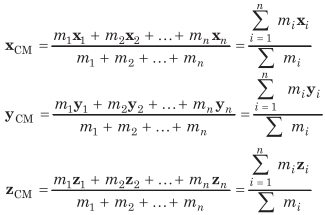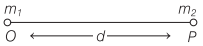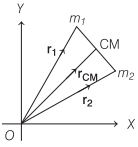# Centre of Mass in Physics | Definition, Examples, Formulas – Rotational Motion

Centre of Mass Definition Physics:
Centre of mass of a system is the point that behaves as whole mass of the system is concentrated on it and all external forces are acting on it. For rigid bodies, centre of mass is independent of the state of the body, i.e. whether it is in rest or in accelerated motion centre of mass will remain same.

We are giving a detailed and clear sheet on all Physics Notes that are very useful to understand the Basic Physics Concepts.

## Centre of Mass in Physics | Definition, Examples, Formulas – Rotational Motion

Centre of Mass of System of n Particles
If a system consists of n particles of masses m1, m2, m3,…,mn having position vectors r1, r2, r3,…, rn then position vector of centre of mass of the system.In terms of coordinates,Centre of Mass of Two Particle System
Choosing O as origin of the coordinate axis.,
(i) Then, position of centre of mass from m1 = $$\frac{m_{2} d}{m_{1}+m_{2}}$$(ii) Position of centre of mass from m2 = $$\frac{m_{1} d}{m_{1}+m_{2}}$$

(iii) If position vectors of particles of masses m1 and m2 are r1 and r2, respectively, then

$$\mathbf{r}_{\mathrm{CM}}=\frac{m_{1} \mathbf{r}_{1}+m_{2} \mathbf{r}_{2}}{m_{1}+m_{2}}$$(iv) If in a two particles system, particles of masses m1 and m2 are moving with velocities v-, and v2 respectively, then velocity of the centre of mass,

$$\mathbf{v}_{\mathrm{CM}}=\frac{m_{1} \mathbf{v}_{1}+m_{2} \mathbf{v}_{2}}{m_{1}+m_{2}}$$

(v) If accelerations of the particles are a! and a2 respectively, then acceleration of the centre of mass,

$$\mathbf{a}_{\mathrm{CM}}=\frac{m_{1} \mathbf{a}_{1}+m_{2} \mathbf{a}_{2}}{m_{1}+m_{2}}$$

(vi) Centre of mass of an isolated system has a constant velocity. It means, isolated system will remain at rest if it is initially at rest or will move with a same velocity, if it is in motion initially.

(vii) The position of centre of mass depends upon the shape, size and distribution of the mass of the body.

(viii) The centre of mass of an object need not to lie with in the object.

(ix) In symmetrical bodies having homogeneous distribution of mass the centre of mass coincides with the geometrical centre of the body.

(x) The position of centre of mass of an object changes in translatory motion but remains unchanged in rotatory motion.

Rotational Motion:
In this portion, we will learn about the rotational motion of the objects. A body moves completely in rotational motion when each particle of the body moves in a circle about a single line. When a force is applied on a body about an axis it causes a rotational motion. The force applied here is called the torque. The axis of the rotation usually goes through the body. Also, learn the two theorems such as parallel axes and perpendicular theorem explained with respect to rotational motion of objects.